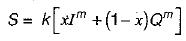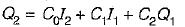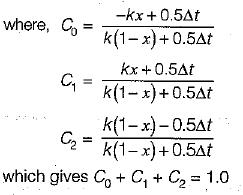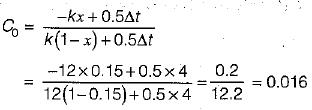Courses

# Flood Routing - 1

## 10 Questions MCQ Test Mock Test Series for Civil Engineering (CE) GATE 2020 | Flood Routing - 1

Description
This mock test of Flood Routing - 1 for Civil Engineering (CE) helps you for every Civil Engineering (CE) entrance exam. This contains 10 Multiple Choice Questions for Civil Engineering (CE) Flood Routing - 1 (mcq) to study with solutions a complete question bank. The solved questions answers in this Flood Routing - 1 quiz give you a good mix of easy questions and tough questions. Civil Engineering (CE) students definitely take this Flood Routing - 1 exercise for a better result in the exam. You can find other Flood Routing - 1 extra questions, long questions & short questions for Civil Engineering (CE) on EduRev as well by searching above.
QUESTION: 1

Solution:
QUESTION: 2

Solution:
QUESTION: 3

### The St Venant equations for unsteady open-channel flow are

Solution:
QUESTION: 4

In routing a flood through a reach, the point of intersection of inflow and outflow hydrographs coincides with the peak of outflow hydrograph

Solution:
QUESTION: 5

Which of the following is a proper reservoir- routing equation?

Solution:
QUESTION: 6

The Muskingum method of flood routing is a

Solution:

In reservoir routing, the storage is a unique function of outflow discharge, S = f(Q).
However, in channel routing the storage is a function of both outflow and inflow discharge and hence a different routing method is needed from muskingum method of hydrological channel routing storage is given by,QUESTION: 7

The Muskingum method of flood routing assumes the storage S is related to inflow rate I and outflow rate Q of a reach as S =

Solution:

For a given channel reach by selecting a routing interval Δt and using the Muskingum equation, the change in storage is,

S2 - S1 = k(xI2 - I1) + 1 - x(Q2 - Q1)
Where suffixes 1 and 2 refer to the conditions before and after the time interval Δt.

QUESTION: 8

The Muskingum method of flood routing gives Q2 = C0I2 + C1I1 + C2Q1. The coefficients in this equation will have values such that

Solution:QUESTION: 9

The Muskingum channel routing equation is written for the outflow from the reach Q in terms of the inflow l and coefficients C0, C1 and C2 as

Solution:
QUESTION: 10

In the Muskingum method of channel routing the routing equation is written as Q2 = C0I2 + C1I1 + C2Q1. If the coefficients K = 12 h and x = 0.15 and the time step for routing Δt = 4 hr, the coefficient C0 is

Solution: